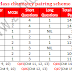This is my 5th post on pairing schemes. Earlier I have posted here the pairing scheme and papers schemes of 9th class all subjects in pdf. I have published separate posts for study schemes of biology and physics of 9th class and also for 10th class of these two subjects. My aim is to provide quality stuff to the students.

## 10th class Chemistry new pairing scheme

For now, I was not sure of the changes in pairing schemes of chemistry for the 10th class. I got news from one of my friends who works in the Lahore board. He gave me the confirm new pairing schemes and now I have decided to share it here. Please share it with your friends.

You may like:
Here is the Paper scheme of 10th class chemistry in picture or image form:

The detailed texts of the pairing scheme are given below:

MCQs = 12 (1 from chapter 11, 12, 14, 16 each and 2 from chapter 9, 10, 13, 15 each)

### Short Questions

Question No. 2 (5/8)
Chapter 9 = 4
Chapter 10 = 4

Question No. 3 (5/8)
Chapter 11 = 3
Chapter 12 = 2
Chapter 13 = 3

Question No. 4 (5/8)
Chapter 14 = 3
Chapter 15 = 2
Chapter 16 = 3

### Long Questions (attempt ANY TWO from three)

Question No. 5
Chapter 9 = one part
Chapter 10 = one part

Question No. 6
Chapter 12 = one part
Chapter 13 = one part

Question No. 7
Chapter 15 = one part
Chapter 16 = one part

You should also see the following posts:
10th class chemistry pairing scheme 2020Reviewed by Saif Ullah ZahidonDecember 22, 2019 Rating: 5